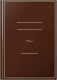Theoretical and Numerical Studies of Reaction-diffusion Systems with Initially Separated Components and for Self-organized Precipitation SystemsAmerican University of Beirut, Computational Science Program, 2011 - 264 Seiten
We present a theoretical and numerical study of some aspects of the coupling of chemical reactions to hydrodynamic diffusion, and it consists of two parts. In the first part, we investigate the dynamics of front propagation in the family of reactions n of A plus m of B yields C with initially segregated reactants in one dimension using hyperbolic reaction-diffusion equations with the mean-field approximation for the reaction rate. This leads to different dynamics than those predicted by their parabolic counterpart. Using perturbation techniques, we focus on the initial and intermediate temporal behavior of the center and width of the front and derive the different time scaling exponents. While the solution of the parabolic system yields a short time scaling as t to the power 0.5 for the front center, width and global reaction rate, the hyperbolic system exhibits linear scaling for those quantities. Moreover, those scaling laws are shown to be independent of the stoichiometric coefficients n and m. The perturbation results are compared with the full numerical solutions of the hyperbolic equations. The critical time at which the hyperbolic regime crosses over to the parabolic regime is also studied. Conditions for static and moving fronts are also derived and numerically validated. The second part of the thesis deals with nucleation and growth in chemical systems. In particular we model and simulate the Liesegang phenomenon in one and two dimensions. A general theory is derived, from which a simplified model is introduced. This results in a set of five coupled non-linear differential equations, the first two describing diffusion and a simple precipitation chemical reaction while the remaining three describe nucleation and growth. We use the control volume method to discretize the equations in space on regular and irregular domains. Finally, the simplified model is extended to include dissolution and polymorphic transition in order to simulate the Liesegang pattern for an experimental nickel hydroxide system.

Was andere dazu sagen -Rezension schreiben

Es wurden keine Rezensionen gefunden.